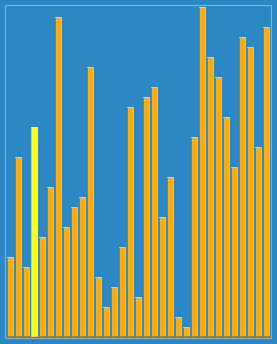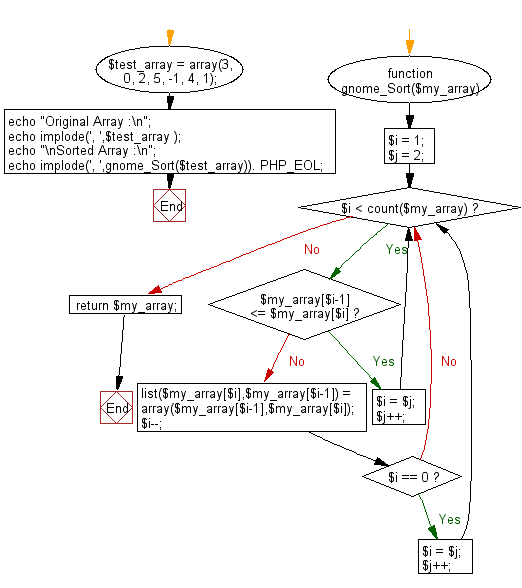﻿ PHP: Sort a list of elements using Gnome sort - w3resource

# PHP Searching and Sorting Algorithm: Gnome sort

## PHP Searching and Sorting Algorithm: Exercise-9 with Solution

Write a PHP program to sort a list of elements using Gnome sort.

Gnome sort is a sorting algorithm originally proposed by Dr. Hamid Sarbazi-Azad (Professor of Computer Engineering at Sharif University of Technology) in 2000 and called "stupid sort" (not to be confused with bogosort), and then later on described by Dick Grune and named "gnome sort".
The algorithm always finds the first place where two adjacent elements are in the wrong order and swaps them. It takes advantage of the fact that performing a swap can introduce a new out-of-order adjacent pair only next to the two swapped elements.

A visualization of the gnome sort :Sample Solution :

PHP Code :

``````<?php
function gnome_Sort(\$my_array){
\$i = 1;
\$j = 2;
while(\$i < count(\$my_array)){
if (\$my_array[\$i-1] <= \$my_array[\$i]){
\$i = \$j;
\$j++;
}else{
list(\$my_array[\$i],\$my_array[\$i-1]) = array(\$my_array[\$i-1],\$my_array[\$i]);
\$i--;
if(\$i == 0){
\$i = \$j;
\$j++;
}
}
}
return \$my_array;
}
\$test_array = array(3, 0, 2, 5, -1, 4, 1);
echo "Original Array :\n";
echo implode(', ',\$test_array );
echo "\nSorted Array :\n";
echo implode(', ',gnome_Sort(\$test_array)). PHP_EOL;
?>
```
```

Sample Output:

```3, 0, 2, 5, -1, 4, 1
Sorted Array :
-1, 0, 1, 2, 3, 4, 5
```

Flowchart :PHP Code Editor:

Have another way to solve this solution? Contribute your code (and comments) through Disqus.

What is the difficulty level of this exercise?

Test your Programming skills with w3resource's quiz.

﻿